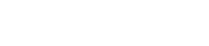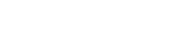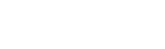AP Calculus BC Review: Polar Functions

Polar functions show up on the AP Calculus BC exam. While this topic shows up in only a handful of problems on any given AP exam, it is worth your while to learn about polar functions in order to maximize your score.

Remember, the higher your score on the AP Calculus BC exam, the better chance you might have to receive college credits!This is a polar bear, not a polar function. Knowing the difference could save your life or at least a few college credits. Image by Ansgar Walk.

Polar Coordinates

When you first learn graphing, you usually plot points (x, y) on a grid by starting at the origin, and then moving x units to the right (or left if x < 0) and y units up (or down if y < 0).

This grid of x– and y-coordinates goes by the fancy name, Cartesian plane.The Cartesian plane

The Cartesian plane is something like a map of city streets. All of the grid lines are straight, equally spaced, and meet at right angles.

The Polar plane

Now imagine you’re at a research station in Antarctica with no streets in sight. All you have for reference is your base camp and a particular direction that you decided to call angle 0, perhaps east on a compass.

Without streets to help you locate points, now you must rely on how far you are from base camp (call that r units), and at what angle to the chosen angle 0 (say, θ radians).

The pair of numbers (r, θ) locates any particular point in the plane. Polar coordinates for a polar research station!Polar graph paper. Each circle represents a constant distance r from the origin. Each line represents an angle θ.

For example, the polar point (3.2, π/2) means that you are exactly r = 3.2 units away from base camp, in the direction of θ = π/2. We should mention here that the angle is always measured counterclockwise from the θ = 0 line. Therefore, if θ = 0 corresponds to east, then π/2 is north.

In this example, it’s easy to see that (3.2, π/2) in polar coordinates would correspond to (0, 3.2) in Cartesian.

Conversion Formulas

There are conversion formulas that help to change polar (r, θ) into Cartesian (x, y), and vice versa.These formulas are based on a little trigonometry.Polar coordinates are related to Cartesian coordinates (x, y) through simple trigonometric formulas (image by P. Wormer)

Plotting in Polar

A polar function is an equation of the form r = f(θ). For every θ-value in the domain of f, you find the corresponding r-value by plugging θ into the function.

It’s really the same idea as plugging in various x-values into a typical (Cartesian) function to find the y-values. The only difference is that for a polar function, your next step would be to plot the polar points, (r, θ).

Example — Graphing a Polar Function

Let’s start with a nice easy polar function, r = 1 + cos θ. First observe that you only need to work out what happens when θ is in the interval [0, 2π]. This is because the cosine function is periodic with period 2π.

Let’s build a table of values. For θ, I typically choose angles that are easy to work with.

θr = 1 + cos θPolar point (r, θ)
02(2, 0)
π/61.866(1.866, π/6)
π/41.707(1.707, π/4)
π/31.5(1.5, π/3)
π/21(1, π/2)
2π/30.5(0.5, 2π/3)
3π/40.293(0.293, 3π/4)
5π/60.134(0.134, 5π/6)
π0(0, π)

Notice that my θ did not go all the way to 2π. We’ll talk about why in a moment. For now though, let’s plot those points.Plot of sample points for r = 1 + cos θ in the domain [0, π]

The general shape should be clear. We’ll draw a smooth curve starting at polar point (2, 0) and connecting the dots in a counterclockwise direction.Now to finish our graph we could list out the values of r for π < θ ≤ 2π repeating the same procedure as above.

Or, we could use our knowledge of the cosine function and save a lot of work!

Remember that cos x has mirror symmetry about x = π. This means that the values of cos x in the interval [π, 2π] will simply rise back up like a mirror image of those in [0, π].

It may be harder to see what happens in the polar plot, but just imagine the curve bouncing back outward as you complete the journey around the full circle. Take a look at the complete graph below.This graph is called a cardioid becausee of its resemblance to a heart.

Graphing by hand is, of course, very time consuming. However if you’re working in a section of the exam that allows a graphing calculator, then I have good news for you.

On most graphing calculators there is setting that puts you into polar mode. Then whatever you graph will be interpreted as a polar function.

Derivatives of Polar Functions

This wouldn’t be calculus unless we started talking about derivatives!

Suppose you want to find the slope of a polar curve. Then the following derivative formula is what you need.Example — Slope of a Polar Function

Consider the cardioid function, r = 1 + cos θ. What is the slope at θ = π/4?

Let’s use the formula to find out. Here, f(θ) = 1 + cos θ.Polar Area Formula

Finally, you can use the following formula to work out the area within a polar curve.Typically on the AP Calculus BC exam, a question may ask for the proper setup of the area integral. On the other hand, if you are in a calculator-permitted section, then you can easily find the area by numerical integration.

Example — Area of the Cardioid

Let’s use our running example and find the area within the cardioid. Remember, f(θ) = 1 + cos θ describes the cardioid for 0 ≤ θ ≤ 2π.Summary

Here are a few points to remember about polar functions.

• This topic only shows up on the AP Calculus BC exam.
• Know how to plot polar points (r, θ) as well as sketch polar functions r = f(θ).
• Know the polar derivative formula (for finding slope).
• Know how to setup the polar area formula.

Improve your SAT or ACT score, guaranteed. Start your 1 Week Free Trial of Magoosh SAT Prep or your 1 Week Free Trial of Magoosh ACT Prep today!Author

•Shaun earned his Ph. D. in mathematics from The Ohio State University in 2008 (Go Bucks!!). He received his BA in Mathematics with a minor in computer science from Oberlin College in 2002. In addition, Shaun earned a B. Mus. from the Oberlin Conservatory in the same year, with a major in music composition. Shaun still loves music -- almost as much as math! -- and he (thinks he) can play piano, guitar, and bass. Shaun has taught and tutored students in mathematics for about a decade, and hopes his experience can help you to succeed!